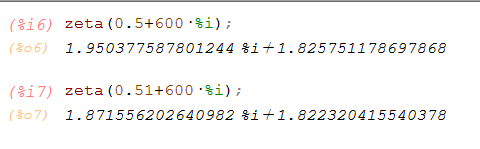# zeta function inaccuracies

Bonnie Saunders shared this question 2 years ago

I have been computing values of the zeta function and getting discrepancies between geogebra and python.

See attach ggb for Geogebra. Below see python computation using mpmath in python 3.6.1

The python results seem better to me. since zeta values seem to agree to better accuracy on the critical line I think the results off that line could be corrected.

>>> from mpmath import *

>>> zeta(.5+600*j)

mpc(real='1.8257511786980125', imag='1.9503775878011067')

>>> zeta(.51+600*j)

mpc(real='1.8223204155405195', imag='1.8715562026408541')

>>>1

Do you know which algorithm Python uses? Maybe we can adapt that1

I do not know python but maxima is open code1

I’m pretty sure Geogebra’s results for values of s off the critical line are wrong. I had an animation where it said ζ(0.128 + 180.9019) was (very close to) 0, which was out of step with Riemann’s hypothesis. When I checked it on a CAS calculator (manually putting in the function, summing to 1000 rather than infinity) the answer was roughly 5.5 + 4.5i. In the animation, the value for zeta(0.5+ti) (I had a slider for t) was a long way from zeta(0.500001 + ti). The values I got for different points on the critical line were synonymous with what I got on my CAS, but none of the others were after t exceeded 50.

I’m not sure what the actual issue is with Geogebra’s algorithm, but you’re right, python would be more accurate.1

We need an algorithm in Java; contributions welcome as always